Next: Kepler's second law Up: Keplerian orbits Previous: Conservation laws

# Plane polar coordinates

We can determine the instantaneous position of our planet in the-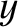plane in terms of standard Cartesian coordinates,,, or plane polar coordinates,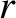,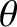, as illustrated in Figure 4.1. Here,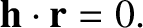and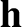. It is helpful to define two unit vectors,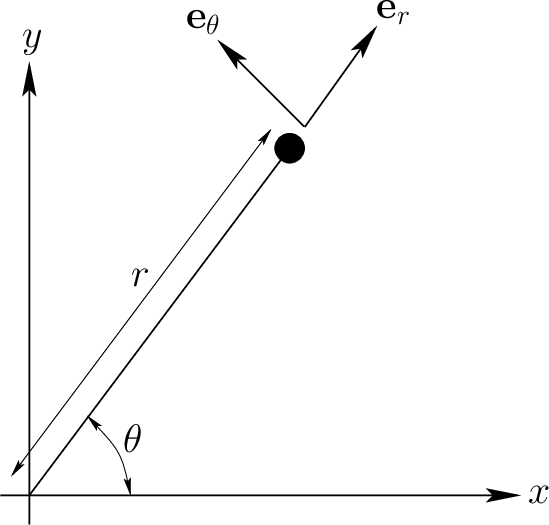and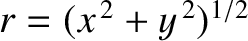, at the instantaneous position of the planet. The first always points radially away from the origin, whereas the second is normal to the first, in the direction of increasing. As is easily demonstrated, the Cartesian components of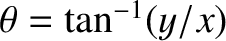and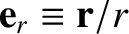are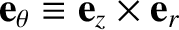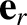(4.8) and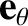(4.9)

respectively.

We can write the position vector of our planet as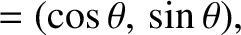(4.10)

Thus, the planet's velocity becomes(4.11)

where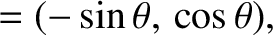is shorthand for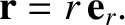. Note thathas a nonzero time derivative (unlike a Cartesian unit vector) because its direction changes as the planet moves around. As is easily demonstrated, by differentiating Equation (4.8) with respect to time,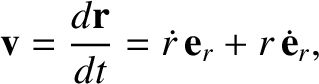(4.12)

Thus,(4.13)

The planet's acceleration is written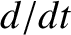(4.14)

Again,has a nonzero time derivative because its direction changes as the planet moves around. Differentiation of Equation (4.9) with respect to time yields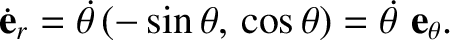(4.15)

Hence,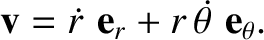(4.16)

It follows that the equation of motion of our planet, Equation (4.2), can be written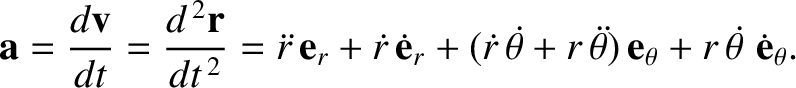(4.17)

Becauseandare mutually orthogonal, we can separately equate the coefficients of both, in the preceding equation, to give a radial equation of motion,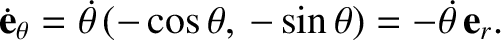(4.18)

and a tangential equation of motion,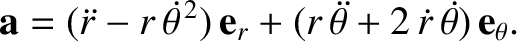(4.19)Next: Kepler's second law Up: Keplerian orbits Previous: Conservation laws
Richard Fitzpatrick 2016-03-31